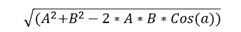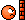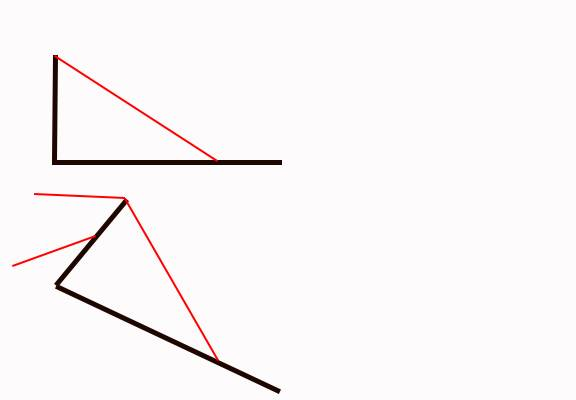# Hinged door lowering below the horizontal

• Ghrober

#### Ghrober

Hi all, This is not a homework question it is trying to get brownie points from my boss.
I have a ramp (mass 2015kg of uniform size) hinged at one end lowered by cables (2) through a sheave 2.7m Vertical. The anchor point on the ramp is 2.7m from the hinge. The overall length of the ramp is 3.3m
What I would like to know is what force is required to lift the door at varying angles?
I have put the information below into a spreadsheet so I can just change (a) thinking it would calculate the force on any angle I entered.
What I know does not work is the formula for C. I have tested the formula against pythag theory(with a =90 degrees) and the length of cable(C) is not the same. Also at angles above 90 degrees the cable length gets shorter?

T=W*L*C/(2*A*B)
W=weight of Door (N)(ton*9.1) 18336.5
L=distance to mass centre of flap(m) 2.6
A=height of cable top from hinge(m) 2.7
B=distance from hinge to anchor on door(m) 2.7C=Length of cable
4.235063
a= angle of open flap to vertical 110
T= 13.8481617 Kn
1.52177601 Te

Any help would be greatly appreciated

I think you'll have to provide a diagram to get good advice.

My interpretation:

There is a 2015 kg ramp of uniform density, 3.3 m long, and hinged at one end. The ramp is raised a lowered by two cables. The cables are attached to opposite sides of the (3.3 m long) edge of the ramp a points 2.7 m from the hinge. Each cable goes over a pulley that is at a vertical distance of 2.7 m [above the level of the hinge?]. The cables are exactly vertical [when the ramp is in an exactly horizontal position? - or when the ramp is lowered so it makes an angle of 110 deg with the upward vertical?].

Find the tension on each cable as a function of the angle the ramp makes [with the upward vertical?].

I think you'll have to provide a diagram to get good advice.

My interpretation:

There is a 2015 kg ramp of uniform density, 3.3 m long, and hinged at one end. The ramp is raised a lowered by two cables. The cables are attached to opposite sides of the (3.3 m long) edge of the ramp a points 2.7 m from the hinge. Each cable goes over a pulley that is at a vertical distance of 2.7 m [above the level of the hinge?]. The cables are exactly vertical [when the ramp is in an exactly horizontal position? - or when the ramp is lowered so it makes an angle of 110 deg with the upward vertical?].

Find the tension on each cable as a function of the angle the ramp makes [with the upward vertical?].

I'll try to send a picture shortly,
But you got most of it. The pulley is 2.7m vertical from the hinge, the cables are never vertical. They form an angle of approx 45 degrees when the ramp is horizontal . The ramp can be lowered approx to 120 degrees from the vertical. I would like to know the tension at any given angle(if possible)

Your formula for the length of the cable is correct.

My first draft is:

Let $\theta = 90 - \alpha$ (degrees) which is the angle of the ramp below the horizontal
Let $\beta$ be the angle of the cable below the horizontal.

$\beta = \arctan( \frac{A + B \sin(\theta)}{B \cos(\theta) }$

$(B \cos(\theta)) [(2T)( \sin(\beta) )] = (L \cos(\theta) ) W$

$2T \sin(\beta) B = LW$

$T = \frac{LW}{ \sin(\beta) B }$

Second draft:

Let $\theta = \alpha - 90$ (degrees) which is the angle of the ramp below the horizontal
Let $\beta$ be the angle of the cable below the horizontal.

$\beta = \arctan( \frac{A + B \sin(\theta)}{B \cos(\theta) }$

$(B \cos(\theta)) [(2T)( \sin(\beta) )] = (L \cos(\theta) ) W$

$2T \sin(\beta) B = LW$

$T = \frac{LW}{ 2 \sin(\beta) B }$

I don't like that design. The hinge location appears illogical. I know that is not helpful, but, it is curious.

•dragoneyes001
Thanks Stephen for all your work.

Chronos,
The ramp is on a boat the cables are raised and lowered by electric motors to allow cargo to be driven on and off the vessel. When the ramp is closed to provides a weather proof door.

Keep in mind that the theoretical calculation can differ from the real life situation. The theoreical calculation assumes the cables are perfectly adjusted so they bear equal weight. The theoretical calculation is for a tension that is necessary to "levitate" the gate at a given angle. To actually raise or lower the gate, it would move with some accelerations, which require additional forces.

Thanks for your comments. I understand what your saying. I was hoping to use the information to confirm that the WLL of the cables are sufficient to raise the door. The winches are also fitted with torque limiters(clutches) that are designed to stop over tensioning of the cables, but still alow the door to be lifted on one cable in the event of a cable/motor failure. I guess the only true way of testing would be to put a load cell on the cable and lift the door?

hi all further to my initial post I have done a calculation using the ramp lowered at a angle of 110 degrees from the vertical using Stephen's formula and the ramp dimensions I supplied and have come up with an answer of 61.101Kn is this correct? if so is this a combined force required over the 2 cables to keep the ramp in levitation ( as Stephen mentioned in his last post). I guess what I really want to know is do I need to divide this by 2 (for each cable) or is it already factored into the equation? THIS IS REALLY OUT OF MY LEAGUEThanks Glenn

Use my second draft. You get the tension on a single cable. I'll get around to checking you numerical answer later.

Some things need to be clarified. You said the ramp is of uniform construction and 3.3 m long, but the distance from the hinge to the center of mass is 2.6 m? If the ramp was uniform, I'd expected the mass center to be halfway from the hinge to the end of the ramp.

The diagram gives the mass of the ramp as 2015 kg. In your first post you show some other numbers for W. Is the correct weight of the ramp (2015 kg)(9.8 Newtons) = 19747.0 Newtons ?

I told a little fib the ramp is not completely uniform but it is 3.3m long. I used the figure of 2.6M as a bit of a guess.
To give you the background, this ramp already has been built and is working on a vessel. It is fitted with 2 x11mm SWR with a WLL 2.3t (min breaking force/5(safety factor)/9.81(convert to ton)-5% (derated due to fittings) and has a history of breaking cables. There are ships drawings that show the ramps fitted with 16mm SWR cables and 11mm cables and I am not sure what cables should be used. Using your formula I was hoping to show, or not show,that the angle of the ramp is exceeding, or not, the WLL of the cables. When I mentioned my concerns to my supervisor he told me that "they already knew that the cables were sufficient to lift the ramp in a straight lift" and believe the issue could be the MBR of the SWR around the sheaves. I don't, so am trying to prove it.

I don't believe the angle of the cables/ramp has ever been taken into consideration. I don't think I'm going to get any arguments from the vessel owners If I say the centre of mass is 2.6 or 1.7m aft of the hinge, but thankyou for being very precise.

The weight of the ramp is 2015kg. That was my mistake I missed the 8 in the tenths column. Should read 2015kg *9.81. I had the data set up in an excel document and it was auto calculating it hence the incorrect mass. It should read as you have stated above 19747n.

Sorry if this all sounds like a rant. I just wanted to give you a bit more of the picture as to what I am using the information for.

Glenn60 degrees beyond vertical would be a problem with that design. because the lifting cable comes too close to in line with the ramp your lifting power will become almost zero.

usually to avoid this especially on boats the pulleys are on top of outrigger posts that also swing outward increasing the angle so the lifting force is maintained

Last edited:I think I've got it. After much studying Stephens formula I have come up with 2.4189T/cable with the ramp @110 Degrees from vertical.
W=weight of Door (N)(ton*9.81) 19767.15
L=distance to mass centre of flap(m) 2.6
A=height of cable top from hinge(m) 2.7
B=distance from hinge to anchor on door(m) 2.7
a= angle of open flap to vertical 110
β=a-90
T=LW/2sin(β)B

T=2.6*19767.15/2*sin(20)*2.7
T= 23730.2798N/1000
T=23.730Kn/9.81
T=2.418ton
A confirmation this is right would be much appreciated

using the ramp lowered at a angle of 110 degrees from the vertical using Stephen's formula and the ramp dimensions I supplied and have come up with an answer of 61.101Kn is this correct?

I get a different tension.

From the c-program
#include <stdio.h>
#include <math.h>

#define A (2.7) /* pulley height in meters */
#define B (2.7) /* hinge to anchor in meters */
#define L (2.6) /* hinge to center of mass in meters */
#define W ((2015.0)*(9.8) ) /* weight of ramp in Newtons */

#define CONV_TO_DEGREES ( 180.0/ M_PI )
#define PI_OVER_TWO (M_PI/2.0)

void main()

{

double theta, beta;
double alpha;
double tension;

alpha = 110.0;

printf("A = %6.3f B = %6.3f L = %6.3f W = %6.3f alpha = %6.3f deg\n",A,B,L,W,alpha);

theta = alpha - 90.0;

printf("theta = %6.3f deg\n",theta);

printf("L*W = %6.3f\n", L*W);

tension = L*W/ (2.0 * sin(beta_rad) * B);

printf("tension = %7.3f Newtons\n", tension);

}

I get the output

A = 2.700 B = 2.700 L = 2.600 W = 19747.000 alpha = 110.000 deg
theta = 20.000 deg
L*W = 51342.200
2.0 * sin(beta_rad)* B = 4.423
tension = 11606.899 Newtons

See if we agree on the intermediate steps.I think I've got it. After much studying Stephens formula I have come up with 2.4189T/cable with the ramp @110 Degrees from vertical.
W=weight of Door (N)(ton*9.81) 19767.15
L=distance to mass centre of flap(m) 2.6
A=height of cable top from hinge(m) 2.7
B=distance from hinge to anchor on door(m) 2.7
a= angle of open flap to vertical 110
β=a-90
T=LW/2sin(β)B

T=2.6*19767.15/2*sin(20)*2.7
T= 23730.2798N/1000
T=23.730Kn/9.81
T=2.418ton
A confirmation this is right would be much appreciated